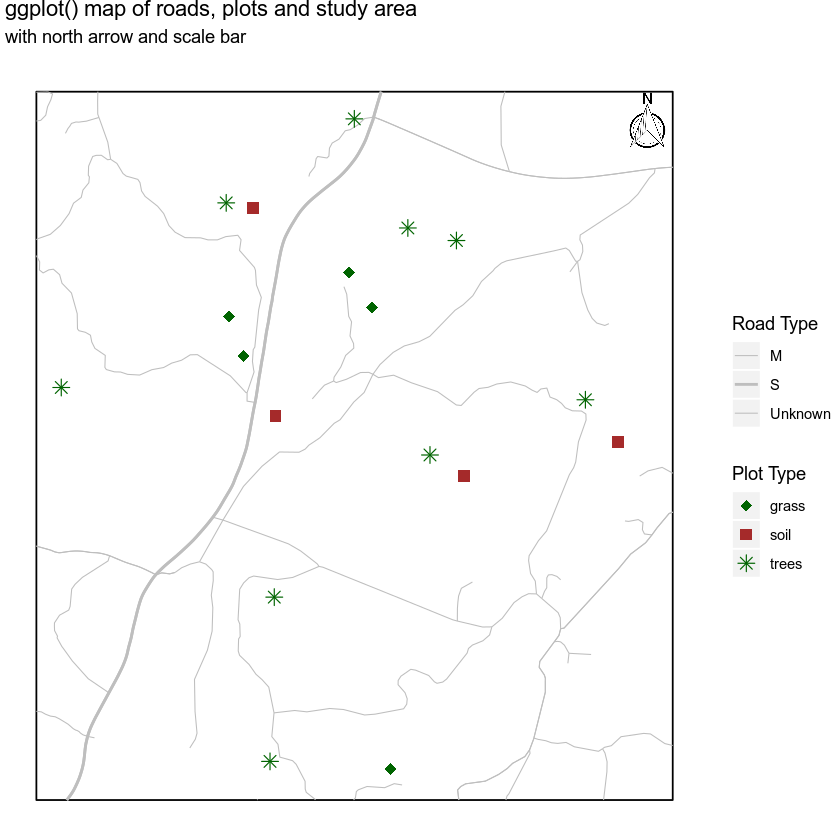# Lesson 2. Maps in R: R Maps Tutorial Using Ggplot

## Learning Objectives

After completing this tutorial, you will be able to:

• Create a map in R using ggplot().
• Plot a vector dataset by attributes in R using ggplot().

## What You Need

You will need a computer with internet access to complete this lesson and the data for week 4 of the course.

## Making Maps with GGPLOT

In the previous lesson, you used base plot() to create a map of vector data - your roads data - in R. In this lesson you will create the same maps, however instead you will use ggplot(). ggplot is a powerful tool for making custom maps. Compared to base plot, you will find creating custom legends to be simpler and cleaner, and creating nicely formatted themed maps to be simpler as well.

However, you will have to convert your data from spatial (sp) objects to data.frames to use ggplot. The process isn’t bad once you have the steps down! Let’s check it out.

Data Tip: If your data attribute values are not read in as factors, you can convert the categorical attribute values using as.factor().

First, let’s import all of the needed libraries.

# load libraries
library(raster)
library(rgdal)
library(ggplot2)
library(broom)
library(RColorBrewer)
library(rgeos)
library(dplyr)
# note that you don't need to call maptools to run the code below but it needs to be installed.
library(maptools)
# to add a north arrow and a scale bar to the map
library(ggsn)
# set factors to false
options(stringsAsFactors = FALSE)


Next, import and explore the data.

# import roads


View attributes of and plot the data.

# view the original class of the TYPE column
class(sjer_roads$RTTYP) ##  "character" unique(sjer_roads$RTTYP)
##  "M" NA  "S" "C"
# quick plot using base plot
main = "Quick plot of roads data")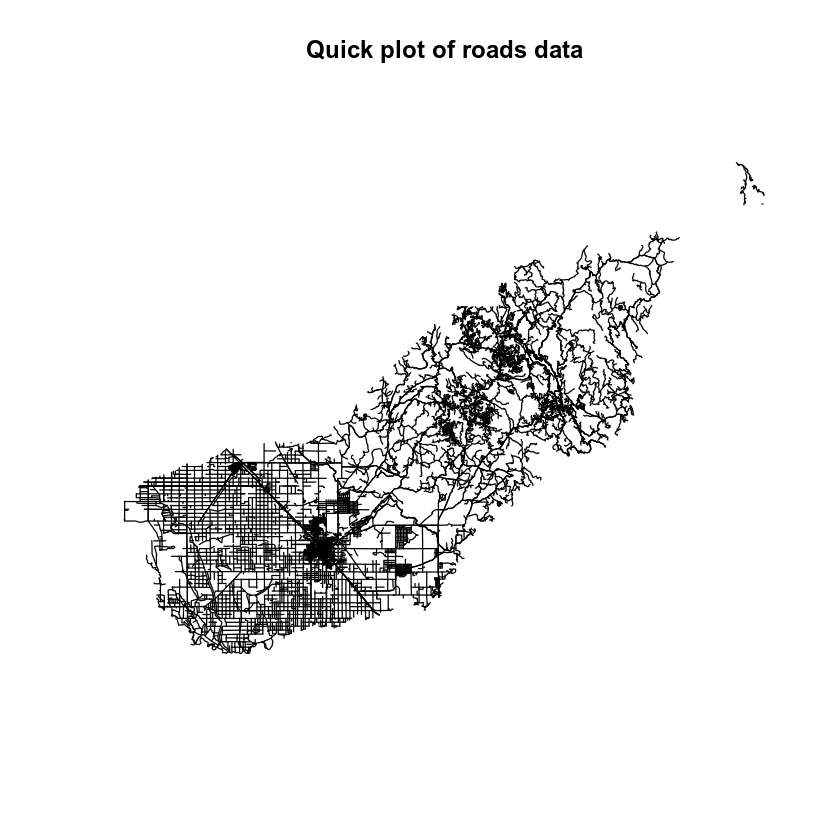It looks like you have some missing values in your road types. You want to plot all road types even those that are NA. Let’s change the roads with an RTTYP attribute of NA to “unknown”.

# set all NA values to "unknown" so they still plot
sjer_roads$RTTYP[is.na(sjer_roads$RTTYP)] <- "Unknown"
unique(sjer_roads$RTTYP) ##  "M" "Unknown" "S" "C"  ## Convert Spatial Data to a data.frame When you’re plotting with base plot(), you can plot spatial sp or raster objects directly without converting them. However ggplot() requires a data.frame. Thus you will need to convert your data. You can convert he data using the tidy() function from the broom package in R. Data Tip: The tidy function used to be the fortify function! The code for the tidy() function is exactly the same as the fortify() code. Below you convert the data by: 1. Calling the tidy() function on your sjer_roads spatial object. 2. Adding an id field to the spatial object data frame which represents each unique feature (each road line) in the data. 3. Joining the table from the spatial object to the data.frame output of the tidy() function. Let’s convert your spatial object to a data.frame. # convert spatial object to a ggplot ready data frame sjer_roads_df <- tidy(sjer_roads, region = "id") # make sure the shapefile attribute table has an id column sjer_roads$id <- rownames(sjer_roads@data)
# join the attribute table from the spatial object to the new data frame
by = "id")


Once you’ve done this, you are ready to plot with ggplot(). Note the following when you plot.

1. The x and y values are long and lat. These are columns that the tidy() function generates from a spatial object.
2. The group function allows R to figure out what vertices below to which feature. So in this case you are plotting lines - each of which consist of 2 or more vertices that are connected.
# plot the lines data, apply a diff color to each factor level)
ggplot() +
geom_path(data = sjer_roads_df, aes(x = long, y = lat, group = group)) +
labs(title = "ggplot map of roads")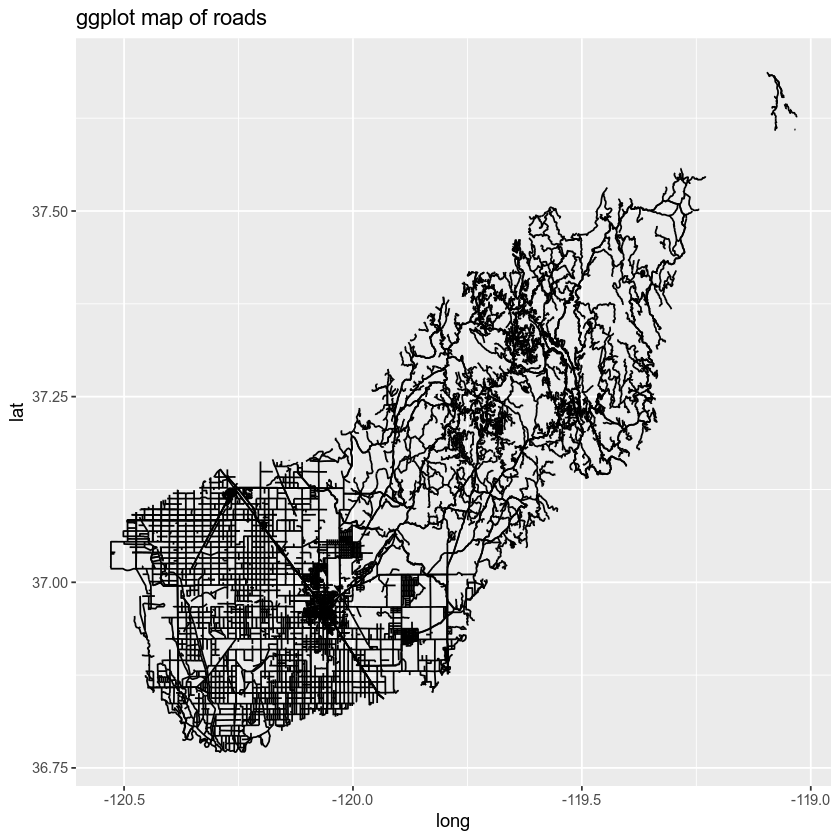You can color each line by type too by adding the attribute that you wish to use for categories or types to the color = argument.

Below you set the colors to color = factor(RTTYP). Note that you are coercing the attribute RTTYP to a factor. You can think of this as temporarily grouping the data by the RTTYP category for plotting purposes only. You aren’t modifying the data you are just telling ggplot that the data are categorical with explicit groups.

# plot roads by attribute
ggplot() +
geom_path(data = sjer_roads_df, aes(x = long, y = lat,
group = group, color = factor(RTTYP))) +
labs(color = 'Road Types', # change the legend type
title = "Roads colored by the RTTP attribute")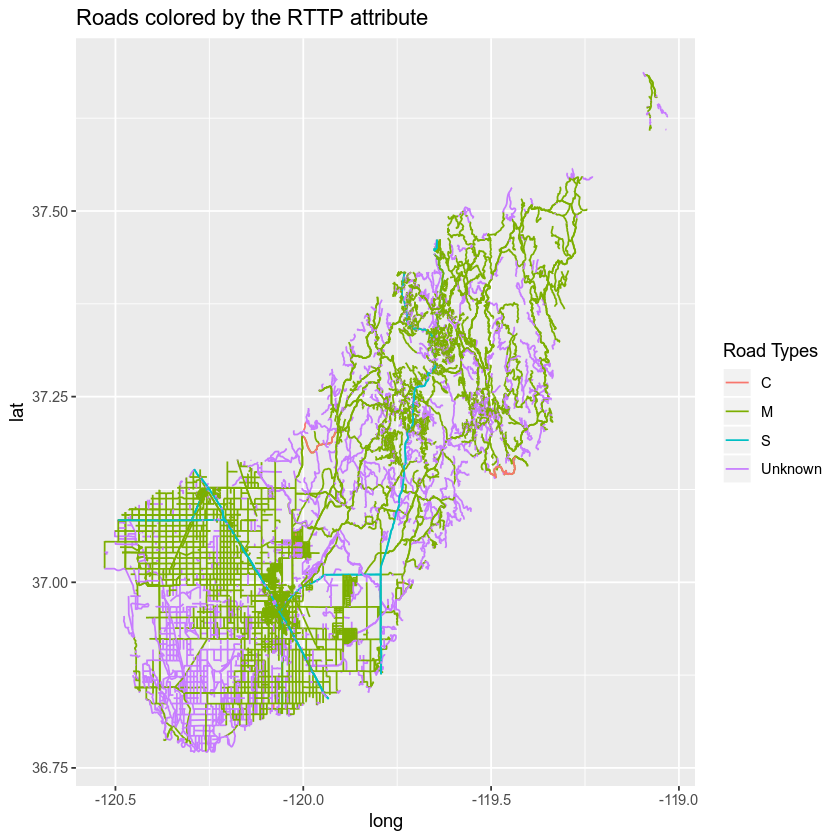You can customize the colors on your map too. Below you do a few things:

1. Figure out how many unique road types you have.
2. Specify the colors that you want to apply to each road type.
3. Finally you plot the data - you will use the scale_colour_manual(values = c("rdType1" = "color1", "rdType2" = "color2", "rdType3" = "color3", "rdType4" = "color4")) to specify what colors you want to use for what attribute value.

Let’s plot your roads data by the RTTYP attribute and apply unique colors.

# count the number of unique values or levels
length(levels(sjer_roads$RTTYP)) ##  0 # create a color palette of 4 colors - one for each factor level road_palette <- c("C" = "green", "M" = "grey40", "S" = "purple", "Unknown" = "grey") road_palette ## C M S Unknown ## "green" "grey40" "purple" "grey" # plot with custom colors # size adjusts the line width ggplot() + geom_path(data = sjer_roads_df, aes(x = long, y = lat, group = group, color=factor(RTTYP))) + scale_colour_manual(values = road_palette) + labs(title = "Madera County Roads ", subtitle = "Colored by road type")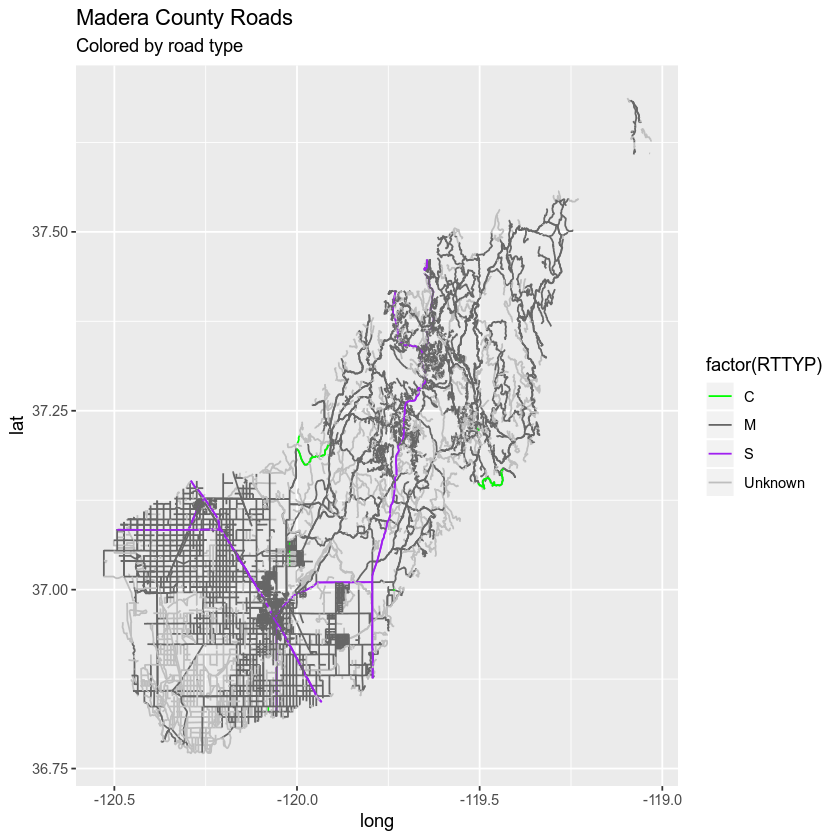Notice that above the colors are applied to each category (C, M, S and Unknown) in order. In this case the order is alphabetical. ### Remove ggplot Axis Ticks Finally you can remove the axis ticks and labels using a theme() element. Themes are used in ggplot() to customize the look of a plot. You can customize any element of the plot including fonts, colors and more! Below you do the following: 1. Remove the x and y axis ticks and label using the theme argument. 2. Remove the x and y labels using the x = and y = arguments in the labs() function. 3. Customize the legend title using labs(color =). Let’s give it a try. # size adjusts the line widht ggplot() + geom_path(data = sjer_roads_df, aes(x = long, y = lat, group = group, color = factor(RTTYP))) + scale_colour_manual(values = road_palette) + labs(title = "Madera County Roads ", subtitle = "Colored by road type", color = "Road type", x = "", y = "") + theme(axis.text = element_blank(), axis.ticks = element_blank())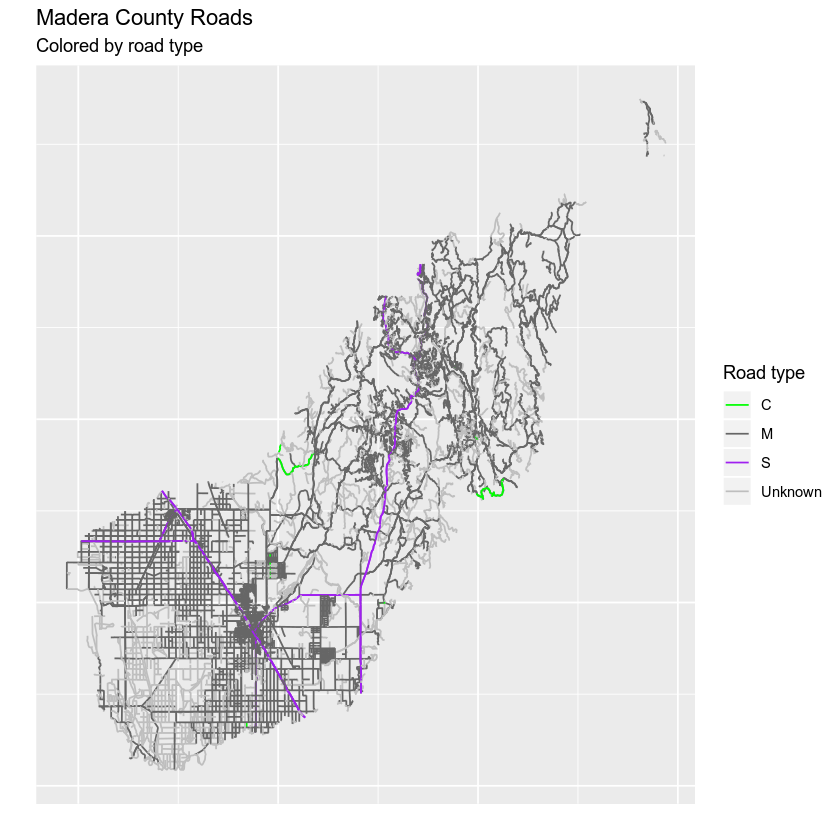Finally you can use coord_quickmap() to scale the x and y axis equally by long and lat values. Data Tip: There are many different ways to ensure ggplot() plots data using x and y axis distances that represent the data properly. coord_fixed() can be used to specify a uniform x and y axis scale. coord_quickmap() quickly adjusts the x and y axis scales using an estimated value of the coordinate reference system that your data are in. coord_map can be used to handle proper projections that you specify as arguments within the coord_map() function. # size adjusts the line width ggplot() + geom_path(data = sjer_roads_df, aes(x = long, y = lat, group = group, color = factor(RTTYP))) + scale_colour_manual(values = road_palette) + labs(title = "Madera County Roads ", subtitle = "Colored by road type", color = "Road type", x = "", y = "") + theme(axis.text = element_blank(), axis.ticks = element_blank()) + coord_quickmap()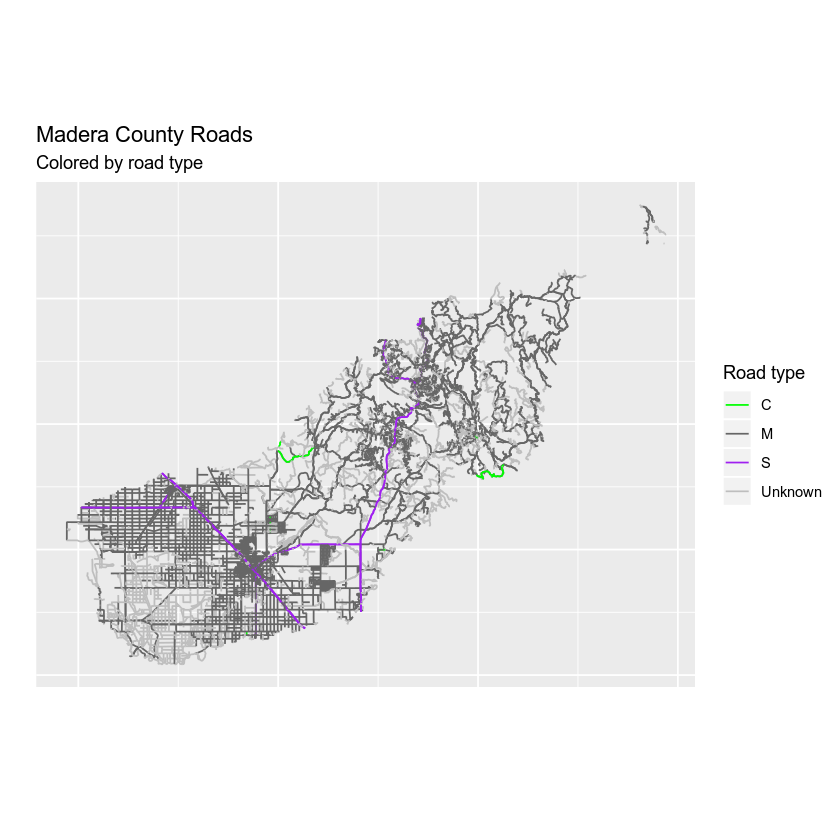### Adjust Line Width You can adjust the width of the lines on your plot using size =. If you use size = 4 with a numeric value (e.g. 4) then you set all of line features in your data to the same size. # size adjusts the line widht ggplot() + geom_path(data = sjer_roads_df, aes(x = long, y = lat, group = group, color = factor(RTTYP)), size = 1.1) + scale_colour_manual(values = road_palette) + labs(title = "Madera County Roads ", subtitle = "With big fat lines!", color = "Road type", x = "", y = "") + theme(axis.text = element_blank(), axis.ticks = element_blank()) + coord_fixed()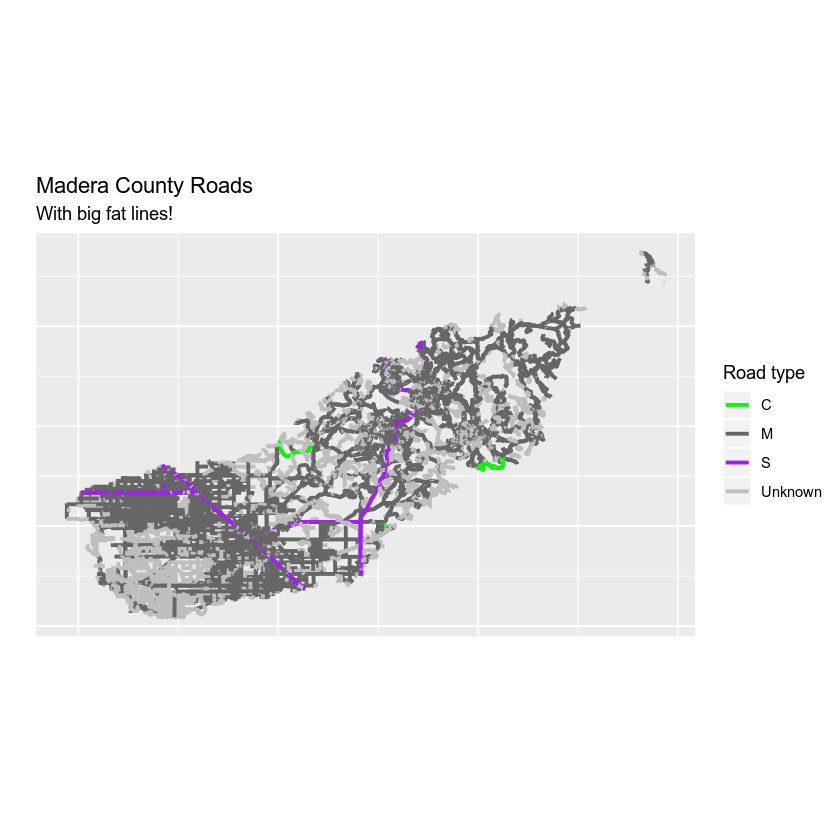### Adjust Line Width by Attribute If you want a unique line width for each factor level or attribute category in your spatial object, you can use a similar syntax to the one you used for colors. Here you use scale_size_manual() to set the line width for each category in the RTTYP attribute. Similar to the colors set above, ggplot() will apply the line width in the order of the factor levels in the data. This is by default alphabetical. scale_size_manual(values = c(.5, 1, 1, .5)) However it’s better to be explicit and set which attribute value should be associated with each line width. Like this: scale_size_manual(values = c("C" = .5, "M" = 1, "S" = 1, "Unknown" = .5)) Note that similar to colors, you have adjusted the lines using two steps 1. You’ve set the size to factor(RTTYP). 2. You’ve assigned the size using the size_scale_manual() function. Let’s see how it looks. # size adjusts the line width # still not working as expected - why does it plot 2 legends # using size for a discrete variable is not advised error -- need to figure this out? ggplot() + geom_path(data = sjer_roads_df, aes(x = long, y = lat, group = group, colour = factor(RTTYP), size = factor(RTTYP))) + scale_size_manual(values = c("C" = .5, "M" = 1, "S" = 1, "Unknown" = .5)) + scale_colour_manual(values = road_palette) + labs(title = "Madera County Roads ", subtitle = "With big fat lines!", color = "Road type", x = "", y = "") + theme(axis.text = element_blank(), axis.ticks = element_blank()) + coord_fixed()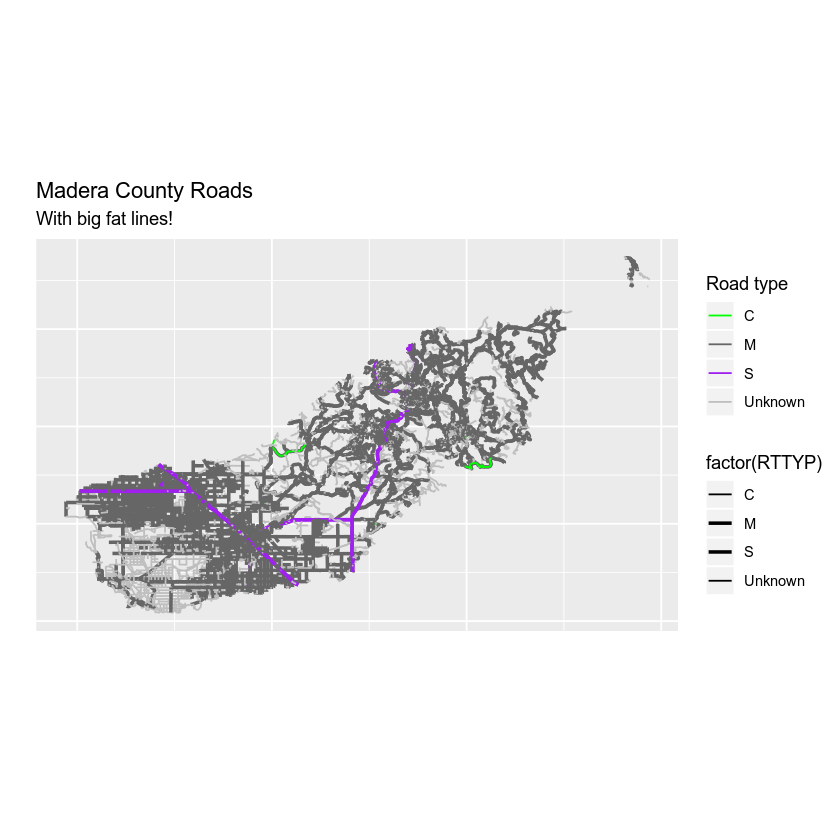### Merge the Legends The map above looks ok but you have multiple legends when you really just want one legend for both color and size. You can merge the legend using the guides() function. Here you specify each legend element that you wish to merge together as follows: guides(colour = guide_legend("Legend title here"), size = guide_legend("Same legend title here")) # size adjusts the line width # still not working as expected - why does it plot 2 legends # using size for a discrete variable is not advised error -- need to figure this out? ggplot() + geom_path(data = sjer_roads_df, aes(x = long, y = lat, group = group, colour = factor(RTTYP), size = factor(RTTYP))) + scale_size_manual(values = c(.5, 1, 1, .5)) + scale_colour_manual(values = road_palette) + guides(colour = guide_legend("Road Type"), size = guide_legend("Road Type")) + labs(title = "Madera County Roads ", subtitle = "With big fat lines!", color = "Road type", x = "", y = "") + theme(axis.text = element_blank(), axis.ticks = element_blank()) + coord_fixed()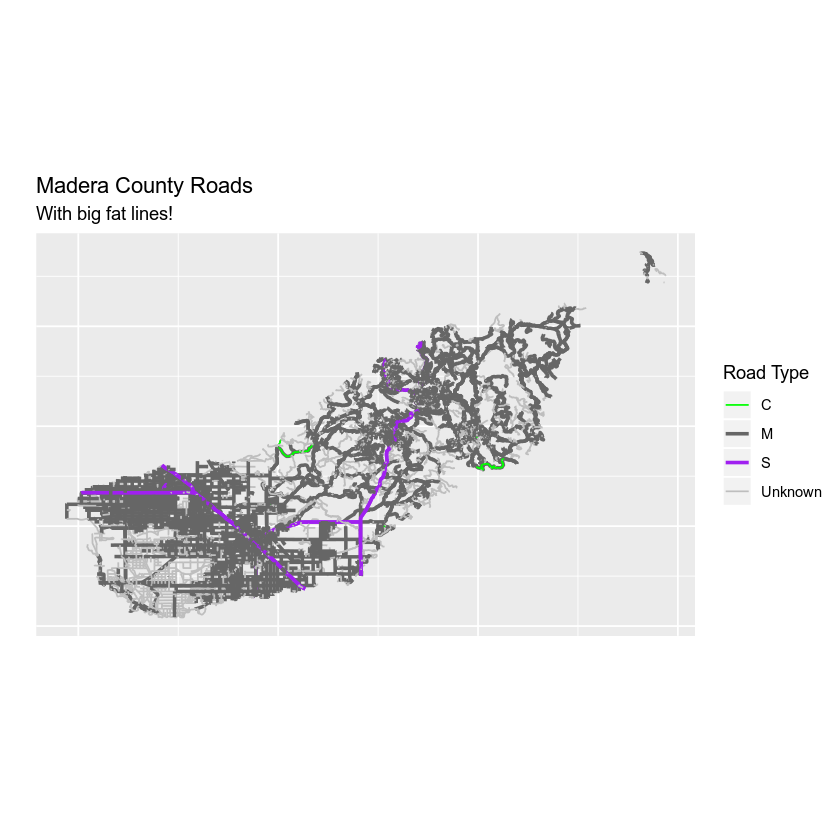But this is ugly, right? Let’s make the line widths a bit thinner to clean things up. # size adjusts the line width # still not working as expected - why does it plot 2 legends # using size for a discrete variable is not advised error -- need to figure this out? ggplot() + geom_path(data = sjer_roads_df, aes(x = long, y = lat, group = group, colour = factor(RTTYP), size = factor(RTTYP))) + scale_size_manual(values = c("C" = .3, "M" = .6, "S" = .6, "Unknown" = .3)) + scale_colour_manual(values = road_palette) + guides(colour = guide_legend("Road Type"), size = guide_legend("Road Type")) + labs(title = "Madera County Roads ", subtitle = "With thinner lines (by attribute)!", color = "Road type", x = "", y = "") + theme(axis.text = element_blank(), axis.ticks = element_blank()) + coord_fixed()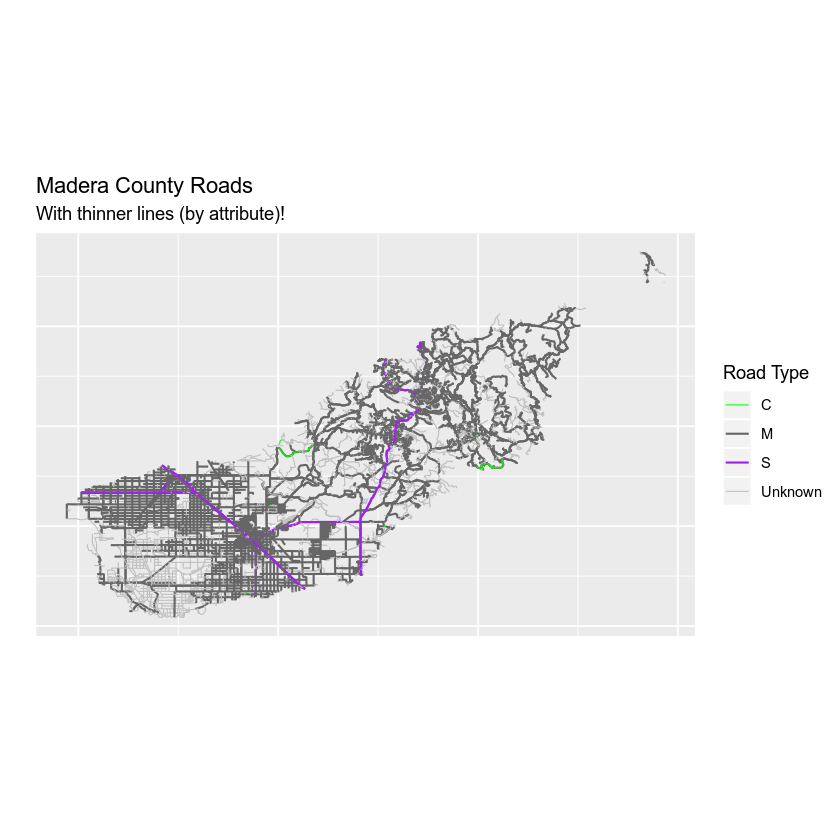## Optional Challenge: Plot Line Width by Attribute Create your own custom map of roads. Adjust the line width and the colors of the roads to make a map that emphasizes roads of value S (thicker lines) and that de-emphasizes roads with an RTTYP attribute value of unknown (thinner lines, lighter color).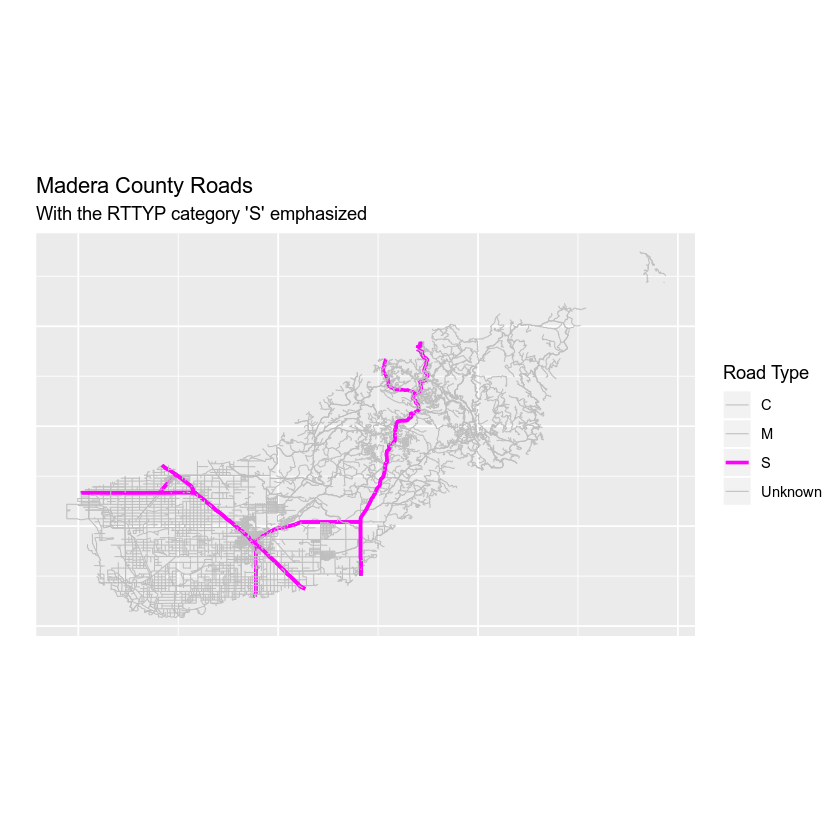## Adding Points and Lines to a Legend Next, let’s add points to your map and and of course the map legend too. You will import the shapefile data/week-04/california/SJER/vector_data/sjer_plot_centroids.shp layer. This data represents study plot locations from your field work in southern California. Let’s import that data and perform any cleanup that is required. # import points layer sjer_plots <- readOGR("data/week-04/california/SJER/vector_data", "SJER_plot_centroids") # given you want to plot 2 layers together, let's check the crs before going any further crs(sjer_plots) crs(sjer_roads) # reproject to lat / long sjer_plots <- spTransform(sjer_plots, crs(sjer_roads))  Next you can tidy() up the data as you did before… or can you? sjer_plots_df <- tidy(sjer_plots, region = "id")  Note that this time you imported point data. You can’t use the tidy function on points data. Instead, you simply convert it to a data.frame using the function as.data.frame. # convert spatial object to a ggplot ready data frame sjer_plots_df <- as.data.frame(sjer_plots, region = "id")  Next, let’s plot the data using ggplot. # plot point data using ggplot ggplot() + geom_point(data = sjer_plots_df, aes(x = coords.x1, y = coords.x2)) + labs(title = "Plot locations")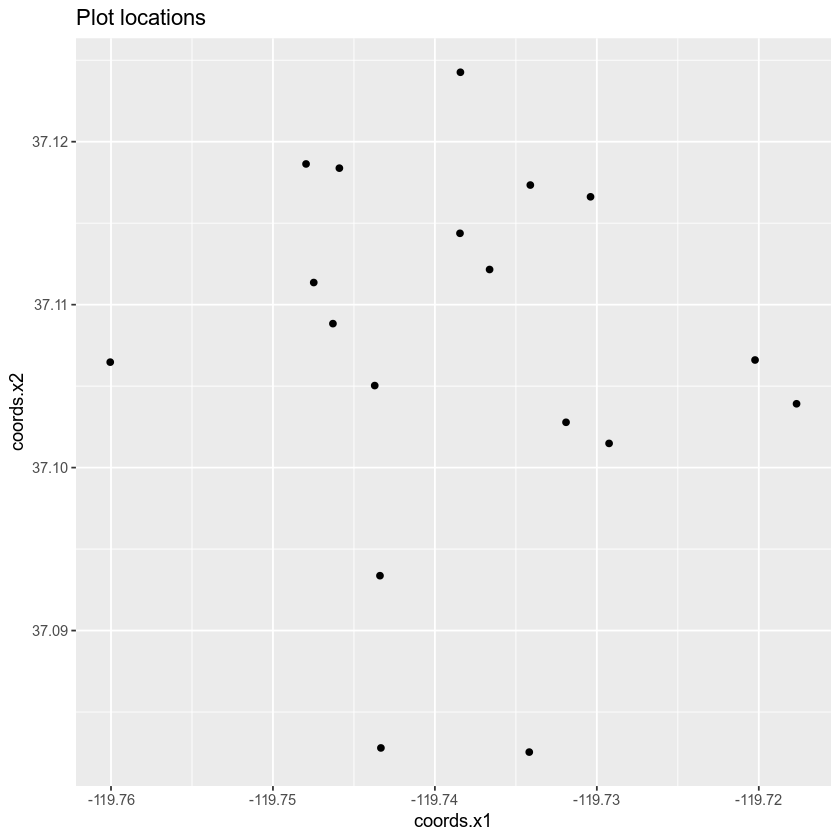Great! You’ve now plotted your data using ggplot. Let’s next combine the roads with the points in one clean map. ## Layering Data in ggplot You can layer multiple ggplot objects by adding a new geom_ function to your plot. For the roads data, you used geom_path() and for points you use geom_point(). Note that you add an addition data layer to your ggplot map using the + sign. # plot lines and points together ggplot() + geom_path(data = sjer_roads_df, aes(x = long, y = lat, group = group), color = "grey") + geom_point(data = sjer_plots_df, aes(x = coords.x1, y = coords.x2))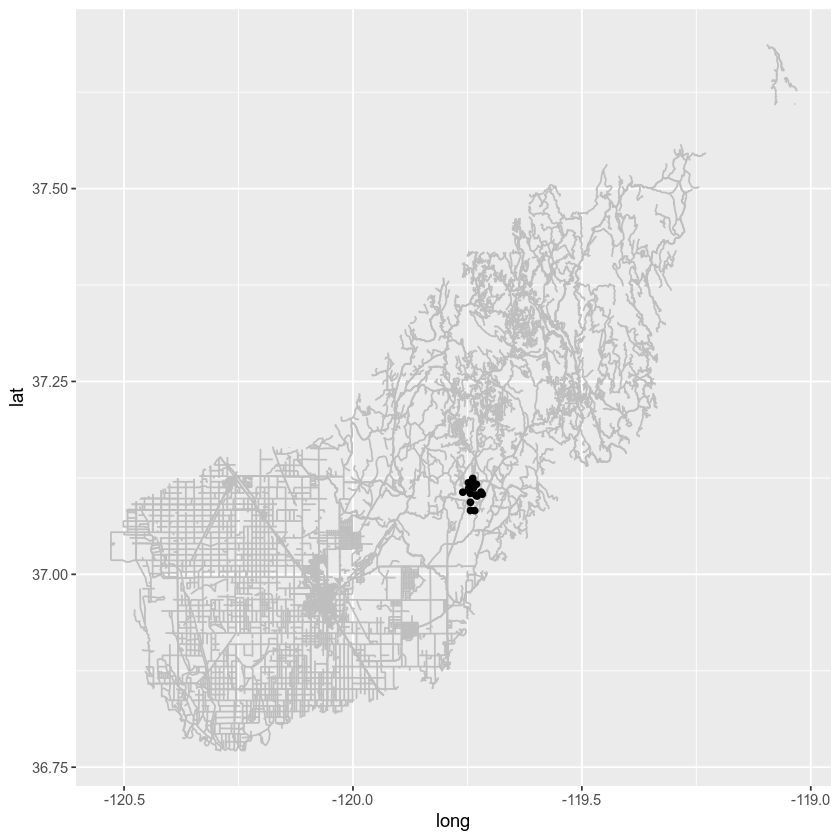Next you have a few options - your roads layer is a much larger spatial extent compared to your plots layer. You could zoom in on your map using coord_fixed() # plot with ggplot adjusting the extent with coord_fixed() ggplot() + geom_path(data = sjer_roads_df, aes(x = long, y = lat, group = group), color = "grey") + geom_point(data = sjer_plots_df, aes(x = coords.x1, y = coords.x2)) + coord_fixed(xlim = c(-119.8, -119.7), ylim = c(37.05, 37.15))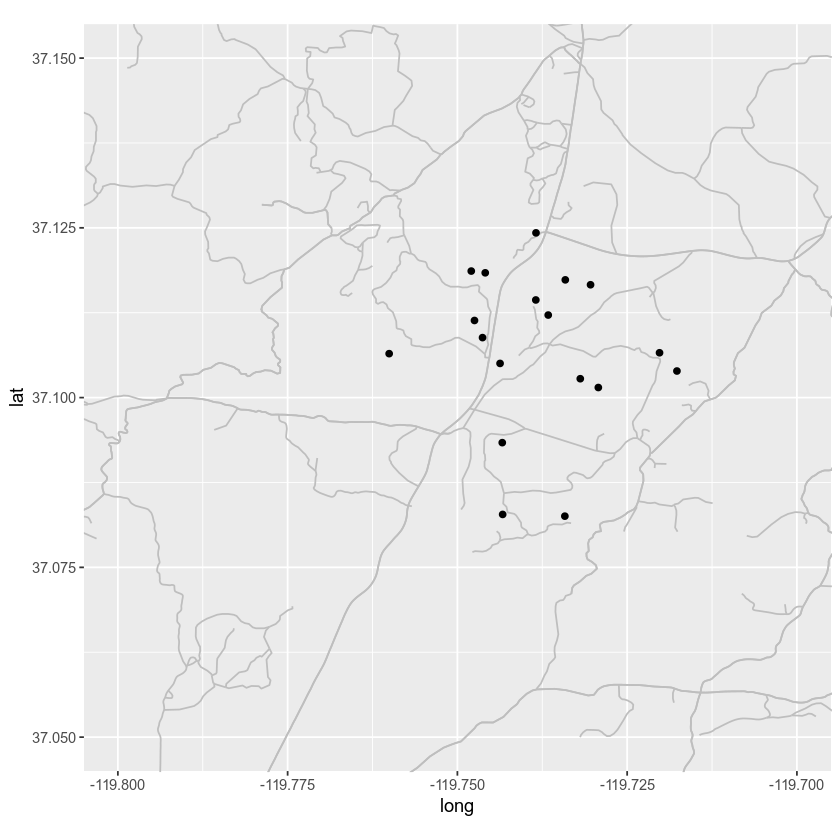## Data Crop vs. Map Zoom A better approach is to crop your data to a study region. That way you aren’t retaining information that you done need which will make plotting faster. Let’s walk through the steps that you did above, this time cropping and cleaning up your data as you go. # import all layers study_area <- readOGR("data/week-04/california/SJER/vector_data/SJER_crop.shp") sjer_plots <- readOGR("data/week-04/california/SJER/vector_data/SJER_plot_centroids.shp") sjer_roads <- readOGR("data/week-04/california/madera-county-roads/tl_2013_06039_roads.shp")  Then explore the data to determine whether you need to clean the data up. # view crs of all layers crs(study_area) ## CRS arguments: ## +proj=utm +zone=11 +datum=WGS84 +units=m +no_defs +ellps=WGS84 ## +towgs84=0,0,0 crs(sjer_plots) ## CRS arguments: ## +proj=utm +zone=11 +datum=WGS84 +units=m +no_defs +ellps=WGS84 ## +towgs84=0,0,0 crs(sjer_roads) ## CRS arguments: ## +proj=longlat +ellps=GRS80 +towgs84=0,0,0,0,0,0,0 +no_defs  It looks like your data are not all in the same coordinate reference system (CRS). Let’s go ahead and reproject the data so they are in the same CRS. Also below you spatially CROP the roads data since you only need road information for your study area. This will also making plotting faster. # reproject the roads to utm sjer_roads_utm <- spTransform(sjer_roads, CRS = crs(study_area)) # assign NA values in the roads layer to "unknown" so you can plot all lines sjer_roads_utm$RTTYP[is.na(sjer_roads_utm$RTTYP)] <- "Unknown" unique(sjer_roads_utm$RTTYP)
##  "M"       "Unknown" "S"       "C"

# crop the roads data to your study area for quicker plotting

# quick plot to make sure the data look like you expect them too post crop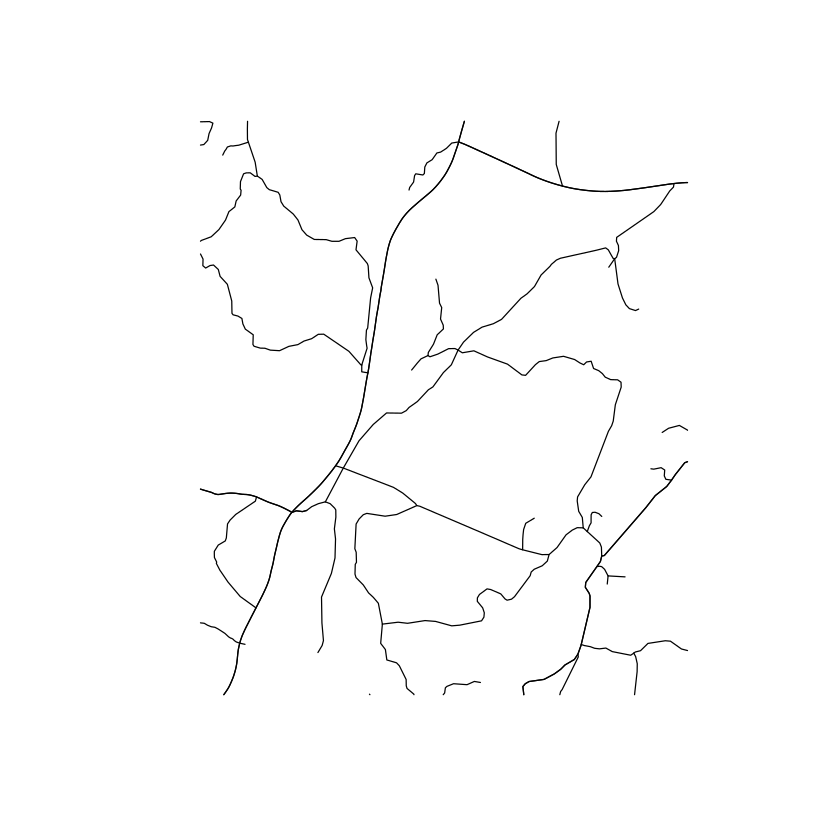# view crs of all layers
crs(study_area)
## CRS arguments:
##  +proj=utm +zone=11 +datum=WGS84 +units=m +no_defs +ellps=WGS84
## +towgs84=0,0,0
crs(sjer_plots)
## CRS arguments:
##  +proj=utm +zone=11 +datum=WGS84 +units=m +no_defs +ellps=WGS84
## +towgs84=0,0,0
## CRS arguments:
##  +proj=utm +zone=11 +datum=WGS84 +units=m +no_defs +ellps=WGS84
## +towgs84=0,0,0


Next, you convert the study_area spatial object to a data.frame so you can plot it using ggplot. In this case you only have one extent boundary for the study area - so you won’t need to add the attributes.

# convert study area data into data.frame
study_area$id <- rownames(study_area@data) study_area_df <- tidy(study_area, region = "id") # convert roads layer to ggplot ready data.frame sjer_roads_df <- tidy(sjer_roads_utmcrop, region = "id") # make sure the shapefile attribute table has an id column so you can add spatial attributes sjer_roads_utmcrop$id <- rownames(sjer_roads_utmcrop@data)
# join the attribute table from the spatial object to the new data frame
by = "id")

# convert spatial object to a ggplot ready data frame - note this is a points layer
# so you don't use the tidy function
sjer_plots_df <- as.data.frame(sjer_plots, region = "id")


Now that you have all of your layers converted and cleaned up you can plot them. Notice that plotting is much faster when you crop the data to only the study location that you are interested in.

# plot using ggplot
ggplot() +
geom_polygon(data = study_area_df, aes(x = long, y = lat, group = group),
fill = "white", color = "black") +
geom_path(data = sjer_roads_df, aes(x = long, y = lat,
group = group, colour = factor(RTTYP))) +
geom_point(data = sjer_plots_df, aes(x = coords.x1,
y = coords.x2), shape = 18) +
labs(title = "GGPLOT map of roads, study area and plot locations")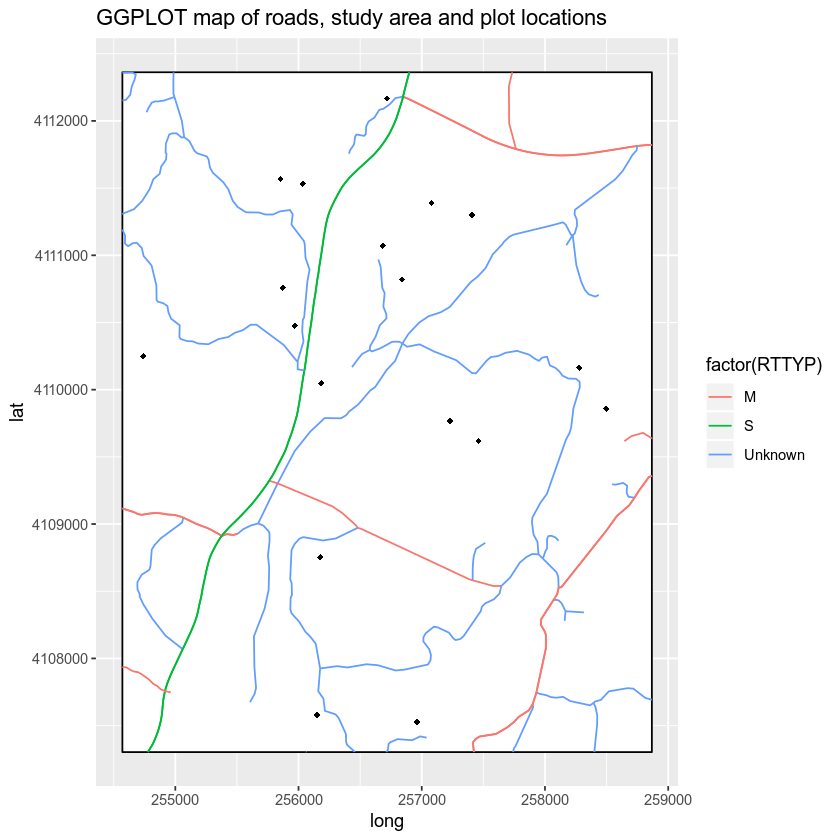Finally, let’s clean up your map even more. That’s apply unique symbols to your plots using the plot_type attribute. Note that it can be difficult to apply unique colors to multiple object types in a ggplot map. Thus you will keep your symbology simple. Let’s make all of the roads the same width and map colors and symbols to the plot locations only. The plot locations are what you want to stand out anyway!

# plot ggplot
ggplot() +
geom_polygon(data = study_area_df, aes(x = long, y = lat, group = group),
fill = "white", color = "black") +
geom_path(data = sjer_roads_df, aes(x = long, y = lat,
group = group, size = factor(RTTYP)), colour = "grey") +
scale_size_manual("Road Type", values = c("M" = .3,
"S" = .8,
"Unknown" = .3)) +
geom_point(data = sjer_plots_df, aes(x = coords.x1,
y = coords.x2, shape = factor(plot_type), color = factor(plot_type)), size = 3) +
scale_color_manual("Plot Type", values = c("trees" = "darkgreen",
"grass" = "darkgreen",
"soil" = "brown")) +
scale_shape_manual("Plot Type", values = c("grass" = 18,
"soil" = 15,
"trees" = 8)) +
labs(title = "ggplot map of roads, plots and study area") +
theme_bw()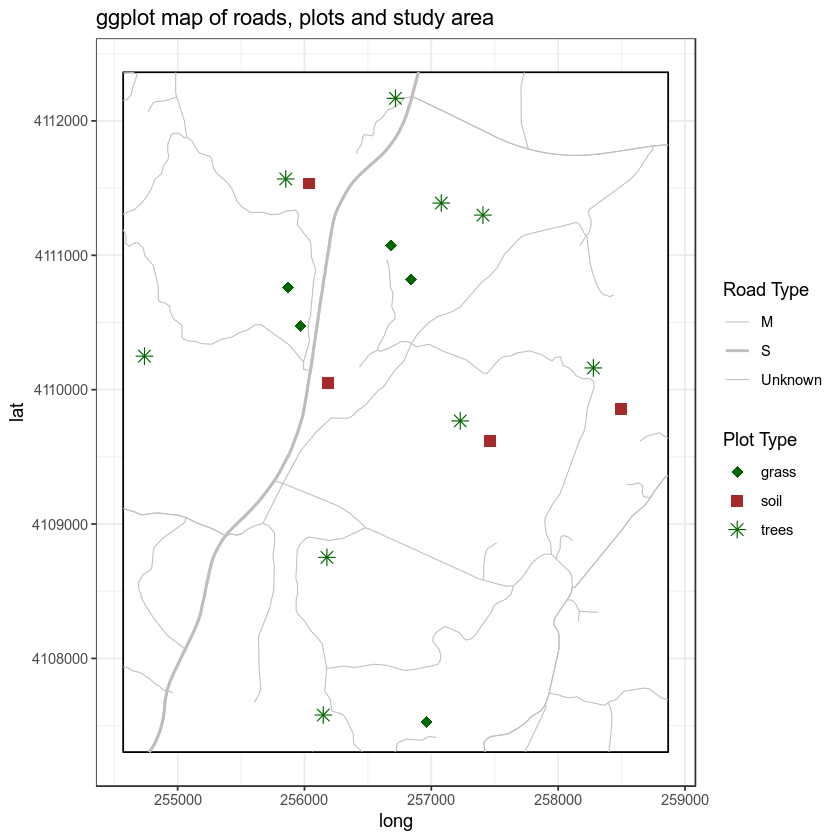Finally, let’s clean up your map further. You can use some of the built in functionality of cowplot to adjust the theme() settings in ggplot.

NOTE: cowplot does the SAME things that you can do with theme() in ggplot. It just simplies the code that you need to clean up your plot! By default it removes the grey background on your plots. However let’s adjust it even further.

# plot ggplot
ggplot() +
geom_polygon(data = study_area_df, aes(x = long, y = lat, group = group),
fill = "white", color = "black") +
geom_path(data = sjer_roads_df, aes(x = long, y = lat,
group = group, size = factor(RTTYP)), colour = "grey") +
scale_size_manual("Road Type", values = c("M" = .3,
"S" = .8,
"Unknown" = .3)) +
geom_point(data = sjer_plots_df, aes(x = coords.x1,
y = coords.x2, shape = factor(plot_type), color = factor(plot_type)), size = 3) +
scale_color_manual("Plot Type", values = c("trees" = "darkgreen",
"grass" = "darkgreen",
"soil" = "brown")) +
scale_shape_manual("Plot Type", values = c("grass" = 18,
"soil" = 15,
"trees" = 8)) +
labs(title = "ggplot map of roads, plots and study area") +
theme_bw()## Adjust ggplot Theme Settings

Finally, you can use a combination of cowplot and ggplot theme() settings to remove the x and y axis labels, ticks and lines. You use background_grid() to remove the grey grid from your plot. Then you use the theme() function to remove:

• the x and y axis text and ticks.
• the axes lines - axis.line.

Let’s see how it looks.

# plot ggplot
ggplot() +
geom_polygon(data = study_area_df, aes(x = long, y = lat, group = group),
fill = "white", color = "black") +
geom_path(data = sjer_roads_df, aes(x = long, y = lat,
group = group, size = factor(RTTYP)), colour = "grey") +
scale_size_manual("Road Type", values = c("M" = .3,
"S" = .8,
"Unknown" = .3)) +
geom_point(data = sjer_plots_df, aes(x = coords.x1,
y = coords.x2, shape = factor(plot_type), color = factor(plot_type)), size = 3) +
scale_color_manual("Plot Type", values = c("trees" = "darkgreen",
"grass" = "darkgreen",
"soil" = "brown")) +
scale_shape_manual("Plot Type", values = c("grass" = 18,
"soil" = 15,
"trees" = 8)) +
labs(title = "ggplot() map of roads, plots and study area",
x = "", y = "") +
cowplot::background_grid(major = "none", minor = "none") +
theme(axis.text.x = element_blank(), axis.ticks.x = element_blank(),
axis.text.y = element_blank(), axis.ticks.y = element_blank(),
axis.line = element_blank())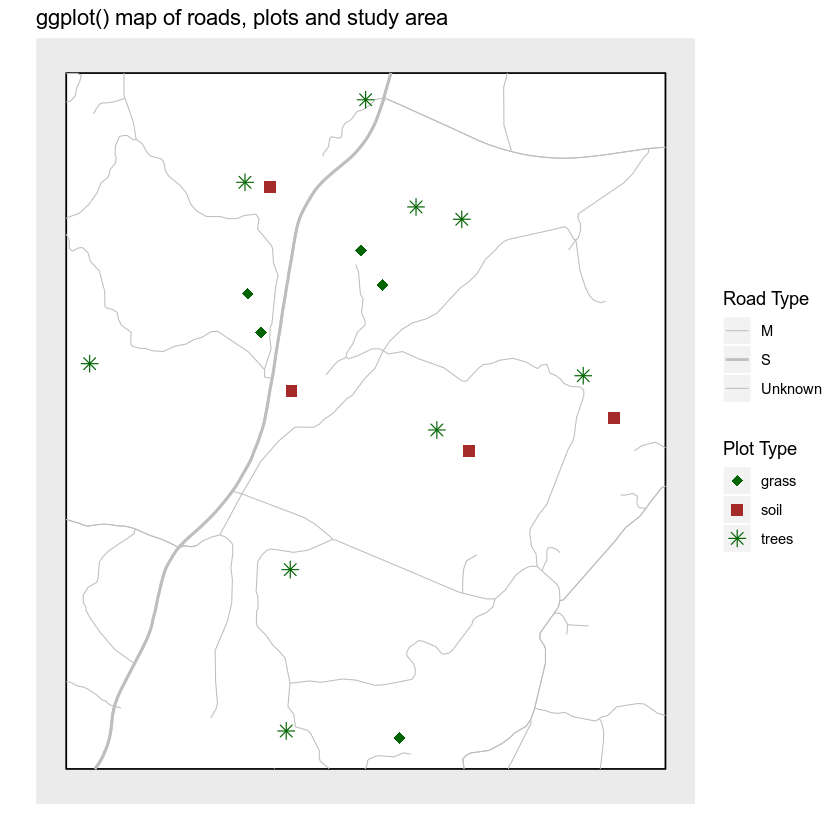## Legends and Scale Bars

You can use the ggsn package to magically add a legend and a scale bar to your final map. You can also use this package to create a “blank” map background - removing all grid background elements. Let’s give it a go.

You can add a scale bar using the scalebar() function. Note that there is also a scalebar function in the raster package so you are explicitly telling R to use the function from the ggsn package using the syntax

ggsn::scalebar()

Also note that there is a bug in the ggsn package currently where the documentation tells us to use dd2km = FALSE for data not in geographic lat / long. However, for this to work, you need to simply omit the argument from function as follows:

For data in UTM meters: ggsn::scalebar(data = sjer_roads_df, dist = .5, location = "bottomright")

For data in geographic lat/long - wgs 84 datum: ggsn::scalebar(data = sjer_roads_df, dd2km = TRUE, model = "WGS84", dist = .5, location = "bottomright")

Below I set the legend location explicitly using the following arguments:

• location: place the scalebar in the bottom right hand corner of the plot
• anchor: this allows me to specify the bottom corner location of my scalebar explicitly
• st.size and st.dist: this allows me to set the size of the text and distance of the text from the scalebar respectively

ggsn::scalebar(data = sjer_roads_df, dist = .5, location = "bottomright", st.dist = .05, st.size = 5, height = .06, anchor = c(x = x_scale_loc, y = (y_scale_loc - 360)))

Finally you can add a north arrow using:

ggsn::north(sjer_roads_df, scale = .08)

You can adjust the size and location of the north arrow as well.

# get x and y location for scalebar

# plot ggplot
ggplot() +
geom_polygon(data = study_area_df, aes(x = long, y = lat, group = group),
fill = "white", color = "black") +
geom_path(data = sjer_roads_df, aes(x = long, y = lat,
group = group, size = factor(RTTYP)), colour = "grey") +
scale_size_manual("Road Type", values = c("M" = .3,
"S" = .8,
"Unknown" = .3)) +
geom_point(data = sjer_plots_df, aes(x = coords.x1,
y = coords.x2, shape = factor(plot_type), color = factor(plot_type)), size = 3) +
scale_color_manual("Plot Type", values = c("trees" = "darkgreen",
"grass" = "darkgreen",
"soil" = "brown")) +
scale_shape_manual("Plot Type", values = c("grass" = 18,
"soil" = 15,
"trees" = 8)) +
labs(title = "ggplot() map of roads, plots and study area",
subtitle = "with north arrow and scale bar",
x = "", y = "") +
# # scalebar isn't quite working just yet.... coming soon!
# ggsn::scalebar(data = sjer_roads_df, dist = .5, location = "bottomright",
#                st.dist = .03, st.size = 4, height = .02, anchor = c(x = x_scale_loc, y = (y_scale_loc - 360))) +
ggsn::north(sjer_roads_df, scale = .08) +
theme(axis.text.x = element_blank(), axis.ticks.x = element_blank(),
axis.text.y = element_blank(), axis.ticks.y = element_blank(),
axis.line = element_blank()) + blank()